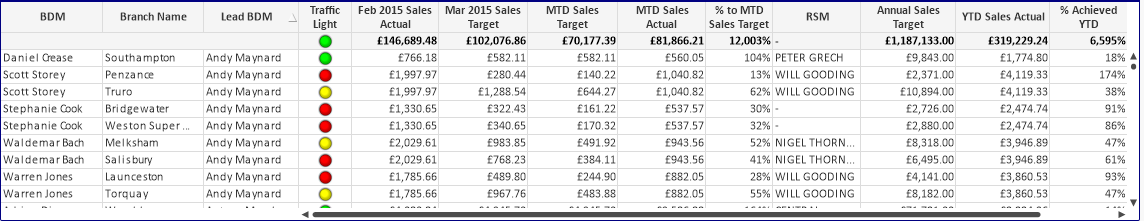New to QlikView

Discussion board where members can get started with QlikView.

HighlightedNot applicable

Calculating only total values in pivot table

Hi experts,

i have the following straight table with some dimensions as BDM, Branch, Lead BDM and some expressions(which is calculated using conditional formulas). all the expressions do not have sum() calculation. so i have made a total of the rows and that total is displayed on top.Now, i need to make another table(pivot or straight table) which should display only the total of the dimensions named Lead BDM.

the problem is the calculation of expressions depends on the particular branch. so if i hide the branch column in pivot table, some values are showing null. but, if i hide branch column in straight table, i get the expected values.

Either of the two is fine i.e., pivot or straight, i have to make a table of total values of Lead BDM alone.

for ex.

i have lead BDM names as

Andy,

Antony,

Nick,

Peter,

Chris,

David, etc....

Now i have to make a table showing the totals alone.

i.e., Total: Lead BDM Andy,

Total: Lead BDM Antony,

Total: Lead BDM Nick, etc... with the same expressions (total alone).

how can i make it.